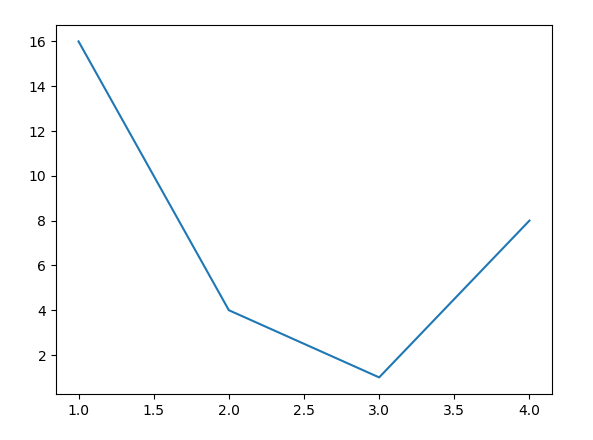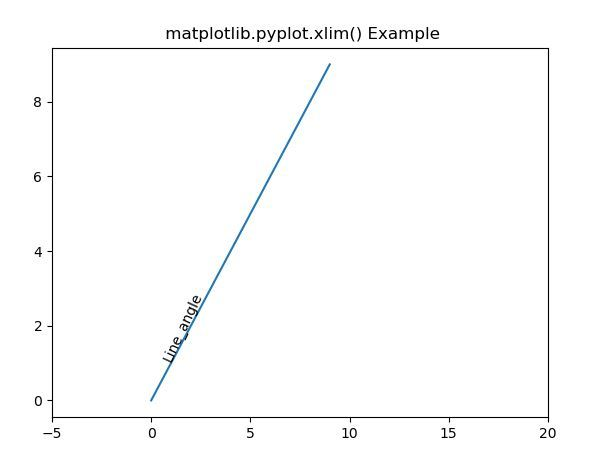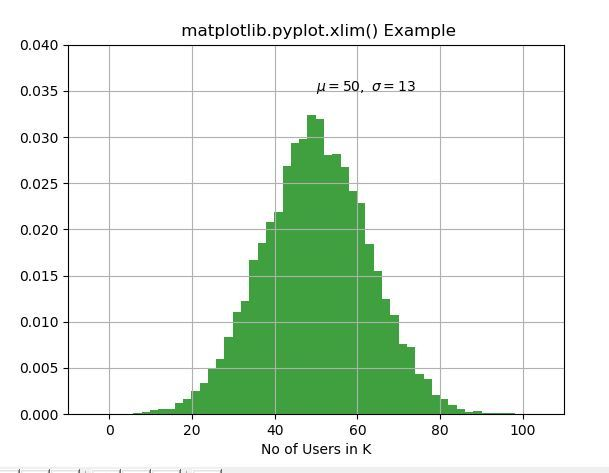# Matplotlib.pyplot.xlim() in Python

• Last Updated : 27 Apr, 2020

Matplotlib is a library in Python and it is numerical – mathematical extension for NumPy library. Pyplot is a state-based interface to a Matplotlib module which provides a MATLAB-like interface. There are various plots which can be used in Pyplot are Line Plot, Contour, Histogram, Scatter, 3D Plot, etc.
#Sample Code

Attention geek! Strengthen your foundations with the Python Programming Foundation Course and learn the basics.

To begin with, your interview preparations Enhance your Data Structures concepts with the Python DS Course. And to begin with your Machine Learning Journey, join the Machine Learning - Basic Level Course

 # sample codeimport matplotlib.pyplot as plt     plt.plot([1, 2, 3, 4], [16, 4, 1, 8]) plt.show()

Output:## matplotlib.pyplot.xlim() Function

The xlim() function in pyplot module of matplotlib library is used to get or set the x-limits of the current axes.
Syntax:

matplotlib.pyplot.xlim(*args, **kwargs)

Parameters: This method accept the following parameters that are described below:

• left: This parameter is used to set the xlim to left.
• right: This parameter is used to set the xlim to right.
• **kwargs: This parameter is Text properties that is used to control the appearance of the labels.

Returns: This returns the following:

• left, right: This returns the tuple of the new x-axis limits.

Below examples illustrate the matplotlib.pyplot.ylim() function in matplotlib.pyplot:

Example-1:

 # Implementation of matplotlib functionimport matplotlib.pyplot as pltimport numpy as np  h = plt.plot(np.arange(0, 10), np.arange(0, 10))plt.xlim([-5, 20])l1 = np.array((1, 1))angle = 65  th1 = plt.text(l1, l1, 'Line_angle',               fontsize = 10, rotation = angle,               rotation_mode ='anchor')  plt.title(" matplotlib.pyplot.xlim() Example")plt.show()

Output:Example-2:

 # Implementation of matplotlib functionimport matplotlib.pyplot as pltimport numpy as np  np.random.seed(9680801)  mu, sigma = 50, 13x = mu + sigma * np.random.randn(10000)  # the histogram of the datan, bins, patches = plt.hist(x, 50,                            density = True,                             facecolor ='g',                             alpha = 0.75)    plt.xlabel('No of Users in K')plt.title('Histogram of IQ')plt.text(50, .035, r'$\mu = 50, \ \ \sigma = 13$')  plt.xlim(-10, 110)plt.ylim(0, 0.04)  plt.grid(True)plt.title(" matplotlib.pyplot.xlim() Example")plt.show()

Output:My Personal Notes arrow_drop_up